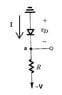# Piece-wise linear diode model

• dalarev

#### dalarev

I am NOT trying to solve this as a homework problem, I'm just trying to dig out basic concepts that I'm missing when using this battery+resistance diode model.

I'm having trouble seeing why, when solving for current, KCL equation does NOT work out as:

1) I= (0-Va)/rd
2) I=(Va-(-5))/R

where Va is the voltage at node a, rd is the diode resistance, and Vd0 is the diode voltage drop.

I understand that the resistors are in series, and there is only one current, so the correct Kirchoff's equation should be:

I=(0-Vd0)/(rd+R)

But each resistor is responsible for a (different) voltage drop. The position of the resistors, even if they are in series, is specific. We can see this by measuring voltages before and after a resistor. Hopefully somebody can provide a fullproof explanation for this.

#### Attachments

•pwlinear.JPG
3 KB · Views: 504
Here's an example directly from the book which very clearly shows the question that I have:

They give us the equation for the current, since there is only one current, and the resistors are in series.

My question is, why is the equation for I NOT:

1) I=(Vdd-Vd0)/R
2) I=(Vd0-0)/rd

Each resistor is responsible for a voltage drop, meaning that it is important where each resistor is located. Where am I going wrong?

#### Attachments

VDD - VD0 gives the sum of the voltage drops across R and rD, hence the given equation for I.

1) I=(Vdd-Vd0)/R
No, because (Vdd-Vd0) is not the voltage across R.

2) I=(Vd0-0)/rd
No, because Vd0 is not the voltage drop across rD

No, because (Vdd-Vd0) is not the voltage across R.

Why not? I'm just following from one voltage source to another, isn't that how KCL works?

It's KVL that we want to use here ... KCL just tells us that the current is the same through each element in the loop.

According to KVL:

VDD - ID*R - VD0 - ID*rD = 0​

You can rearrange that to get

VDD - VD0 = ID*R + ID*rD

Note the right-hand-side has the voltages across both resistors.

Thanks for that, made me realize something I didn't recall:

Consider the entire voltage across the diode, first; then, KVL becomes:

I=(-vd-(-5))/R

from node a to Vss. Now, realizing that for this diode model, the voltage across the diode, (variable) vd:

vd=Vd0+I*rd

where Vd0 is the intrinsic threshold voltage (constant).

Substituting in the original equation for I and playing around with some algebra leads us to:

I=(-Vd0+5)/(R+rd)

which I'm hoping is the right answer. Thanks for the refresher.

Looks good, assuming -V = -5V in your first circuit.